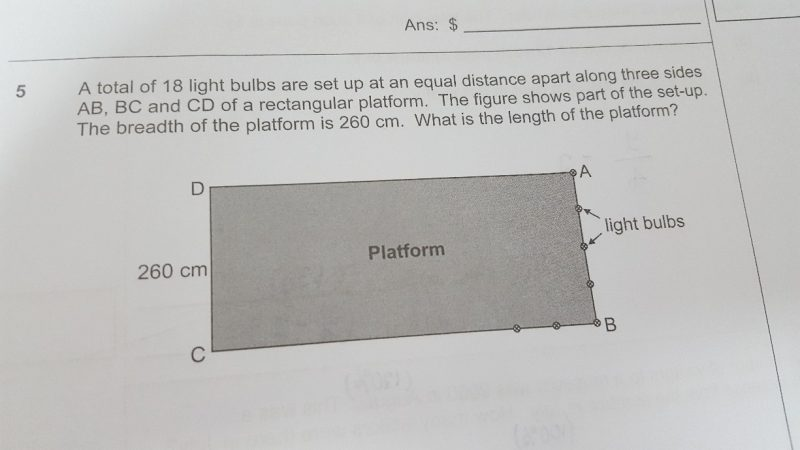# QuestionTotal number of bulbs on AB = 5.

Total number of bulbs on CD = 5.

Total number of bulbs on CB = 18 – 5 (being bulbs on AB) – 5 (being bulbs on CD) + 2 (since the corner bulbs repeat on CB) = 10.

Length of CD = AB = 260cm.

Distance between each bulb = 260 / 4 (being the number of equal distance spaces between 2 bulbs on AB) = 65cm.

Length of platform = distance between each bulb * number of equal distance spaces between 2 bulbs on CB = 65 * 9 = 585cm.

0 Replies 1 Like